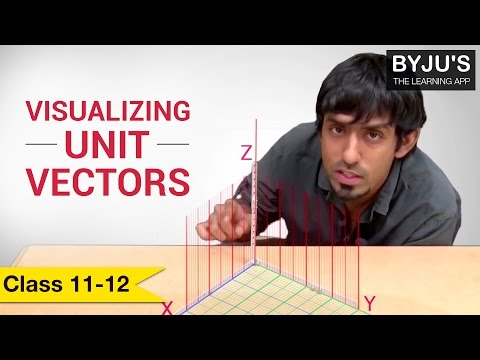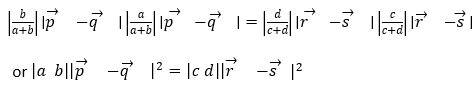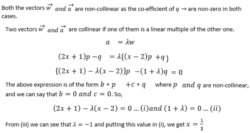# Fundamental Theorem of Vectors

Vectors are defined by magnitude and direction. Vectors can be define as, the line segment AB is a vector which is denoted by $\vec a$ and is read as vector a. Point A is called the initial point from where AB starts, point B is called the terminal point where AB ends and the distance between A and B is called as magnitude or length of the vector $\vec a$ and is denoted by $|\vec a|$. The arrow indicates the direction of the vector.

Position Vectors:

The position vector of a point P(x,y,z) with respect to origin O (0,0,0) is drawn. Its magnitude will be given by|OP| = $\sqrt{x^2+y^2+z^2}$ and the vector $\vec {OP}$ will have O and P as its initial and terminal points, respectively. This vector denotes the position vector of the point P with respect to O.

## Types of Vectors

• Zero Vector: A vector which has got the same initial and ending points. It is denoted by $\vec O$
• Unit Vector: A vector with its magnitude equal to one is called a unit vector. Thus, $\hat i$ is a unit vector of I, where $|\hat i|$ = 1.
• Co-initial vectors: When two or more vectors have the same initial points
• Equal Vectors: Two vectors are equal when they have same magnitude and direction. The initial points doesn’t matter here. They may be represented as: $\vec b$ = $\vec a$
• Negative of a Vector: A vector whose magnitude is the same but direction is opposite to the original vector say $\vec PQ$ is called a negative vector of $\vec O$ i.e. $\vec QP$ = – $\vec PQ$
• Free Vectors: The vectors whose initial points are not fixed.
• Parallel Vectors: The vectors which may have different magnitude but all should have the same or opposite direction are called parallel vectors.
• Collinear Vectors: Vectors which may have same direction or are parallel or anti-parallel. As magnitudes can vary, we can find some scalar vector λ for which $\vec a = \lambda \vec b$.
• Non-collinear Vectors: Two vectors acting in different directions are called non-collinear vectors or independent vectors. Though $\vec a$ and – $\vec b$ have same magnitude, but we can’t express $\vec a$ or – $\vec b$ in terms of one another. Two non-collinear vectors describe a plane.
• Co-planar Vectors: Two parallel vectors or non-collinear vectors are always co-planar to one another. Usually, more than two vectors if they lie within the same plane, they are called co-planar vectors.

## Important Fundamental Theorems of Vectors

In Two Dimensions:

If there are two non-zero or non-collinear vectors $\vec p$ and $\vec q$, then any vector $\vec x$ which lies in the plane of $\vec p$ and $\vec q$ can be written as a linear combination of $\vec p$ and $\vec q$. We can also write this as there exists L and M ϵ R such that L *$\vec p$ + M * $\vec q$ = $\vec x$.

This also proves that if $L_1 \vec p + M_1 \vec q = L_2 \vec p + M_2 \vec q$ exists, then we can write

L1=L2 and M1=M2

In Three Dimensions:

If there are three non-zero or non-collinear vectors $\vec p, \vec q \ and \ \vec r$ then any vector $\vec x$ which lies in the plane of $\vec p, \vec q \; and \; \vec r$ can be written as a linear combination of $\vec p, \vec q \; and \; \vec r$ We can also write this as there exists L, M ad N ϵ R such that

$L \vec p + M \vec q + N \vec r = \vec x$.

This also proves that if $L_1 \vec p + M_1 \vec q + N_1 \vec r = L_2 \vec p + M_2 \vec q + N_2 \vec r$ exists, then we can write L1 = L2, M1 = M2 and N1 = N2

Theorem 1:

If $\vec a \; and \; \vec b$ be two non-collinear vectors, then every vector $\vec i$ which is co-planar with $\vec a \; and \; \vec b$ can be expressed in one and only one combination in the form of $x \vec a + y \vec b= \vec i$ where x and y are scalar components of the respective vectors.

If two vectors are perpendicular to each other, then the vectors can be supposed to be drawn along the X-axis and Y-axis respectively. If the unit vectors along the two vectors is represented by $\hat{i}$ respectively, then we can write that

$\vec t = x \vec i + y \vec j$

Theorem 2:

If $\vec a, \vec b \; and \; \vec c$ are non-coplanar vectors, then any vector $\vec {i}$ can be uniquely expressed as a linear combination $\vec i = x \vec a + y \vec b + z \vec c$ where x, y and z are scalar components of the respective vectors.

Theorem 3:

If vectors $\vec a = a_1 \hat i + a_2 \hat j + a_3 \hat k$;

$\vec b = b_1 \hat i + b_2 \hat j + b_3 \hat k$ and

$\vec c = c_1 \hat i + c_2 \hat j + a_3 \hat k$

are co-planar, then

$\begin{vmatrix} a_1 &a_2 &a_3 \\ b_1& b_2 &b_3 \\ c_1& c_2 &c_3 \end{vmatrix}$

Notes:

1. Three points with position vectors $\vec a, \vec b \; and \; \vec c$ are collinear if and only if there exist scalars like x, y and z all of which is not equal to zero such that
$x \vec{a} + y \vec{b} + z \vec{c} = 0$ and x + y + z = 0

1. Four points with position vectors $\vec a, \vec b, \vec c\; and\; \vec d$ are coplanar if and only if there exist scalars like x, y, z and w (sum of any two is not equal to zero) such that
$x \vec{a} + y \vec{b} + z \vec{c} + w \vec{d} = 0$ and x + y + z + w = 0

### Important terms used in theory of vectors:

Linear Combination:

A vector $\vec x$ is said to be a linear combination of vectors $\vec {a_1}, \vec {a_2}, \vec {a_3},…….,\vec {a_n}$ in a way that there exists scalar $m_1, m_2, m_3,……., m_n$ and we can write $\vec x = \vec{a_1} m_1+ \vec{a_2} m_2+ \vec{a_3} m_3+…+\vec{a_n} m_n$

Linear Independence:

A system of vectors $\vec {a_1}, \vec {a_2}, \vec {a_3},…….,\vec {a_n}$ are said to be linearly independent

If $\vec x = \vec{a_1} m_1+ \vec{a_2} m_2+ \vec{a_3} m_3+…+\vec{a_n} m_n = 0$ which means that

m1=m2=m3 =…=mn=0

Condition:

• A pair of non-collinear vectors is linearly independent.
• A triad of non-coplanar vector is linearly independent.

Linear Dependence:

A set of vectors $\vec {a_1}, \vec {a_2}, \vec {a_3},…….,\vec {a_n}$ is said to be linearly dependent such that scalars $m_1, m_2, m_3,……., m_n$ exists such that all of the scalars are not equal to zero and

$\vec{a_1} m_1+ \vec{a_2} m_2+ \vec{a_3} m_3+…+\vec{a_n} m_n = 0$

Condition:

• A pair of collinear vectors is linearly independent.
• A triad of coplanar vector is linearly independent.

## Related Video Lesson:## Solved Questions

Question 1: Points P ($\vec{p}$), Q ($\vec{q}$), R ($\vec{r}$) and S ($\vec{s}$) are related as $a \vec{p} + b \vec{q}+ c \vec{r} + d \vec{s}=0$), and a + b + c + d = 0 (where a, b, c, d are scalars and sum of any two is not zero). Prove that if P, Q, R and S are concyclic then

$|ab||\vec p – \vec q|^2 = |cd| |\vec r – \vec s|^2$

From the question given, it is understood that P, Q, R and S are coplanar.

Now they are concyclic also.

So, we can write

PO x QO = RO x SO

OrQuestion 2: Vectors $\vec p$ and $\vec q$ are non-collinear. Find for what value of y vector $\vec w = (x – 2)\vec p + \vec q$ and $\vec a = (2x + 1)\vec p – \vec q$ are collinear?